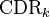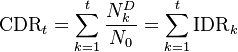# Cumulative Default Rate

## Definition

The term Cumulative Default Rate is used in the context of multi-period credit risk analysis to denote the empirical (or modelled) default rate observed in a certain portfolio as it cumulates (aggregates) up to a final point in time.

The cumulative default rate up to time period k is denoted$\mbox{CDR}_{k}$ and can be considered as the integral (sum) of the Incremental Default Rate

## Formula

The cumulative default rate during period k, given an initial count of N0 and incremental default rate NtD$\mbox{CDR}_{t} = \sum_{k=1}^{t} \frac{N^{D}_k}{N_0} = \sum_{k=1}^{t} \mbox{IDR}_{k}$